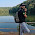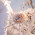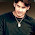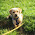# Time, Speed and Distance: Concepts and Problems

Today I am going to discuss a very important topic Time, Speed and Distance. This concept is used extensively for questions related to different areas of CAT, GMAT and Bank exams. For example boats and streams, trains, clocks etc.

## Concepts

1) There is a relationship between speed, distance and time:

Speed = Distance / Time OR

Distance = Speed* Time

2) Average Speed = 2xy / x+y

where x km/hr is a speed for certain distance and y km/hr is a speed at for same distance covered.

**** Remember that average speed is not just an average of two speeds i.e. x+y/2. It is equal to 2xy / x+y

3) Always remember that during solving questions units must be same. Units can be km/hr, m/sec etc.

**** Conversion of km/ hr to m/ sec and m/ sec to km/ hr

x km/ hr = (x* 5/18) m/sec i.e. u just need to multiply 5/18

Similarly, x m/sec = (x*18/5) km/sec

4) As we know, Speed = Distance/ Time. Now, if in questions Distance is constant then speed will be inversely proportional to time i.e. if speed increases ,time taken will decrease and vice versa.

## Time and Distance Problems

Problem 1: A man covers a distance of 600m in 2min 30sec. What will be the speed in km/hr?

Solution: Speed =Distance / Time
⇒ Distance covered = 600m, Time taken = 2min 30sec = 150sec
Therefore, Speed= 600 / 150 = 4 m/sec
⇒ 4m/sec = (4*18/5) km/hr = 14.4 km/ hr.

Problem 2: A boy travelling from his home to school at 25 km/hr and came back at 4 km/hr. If whole journey took 5 hours 48 min. Find the distance of home and school.

Solution: In this question, distance for both speed is constant.
⇒ Average speed = (2xy/ x+y) km/hr, where x and y are speeds
⇒ Average speed = (2*25*4)/ 25+4 =200/29 km/hr
Time = 5hours 48min= 29/5 hours
Now, Distance travelled = Average speed * Time
⇒ Distance Travelled = (200/29)*(29/5) = 40 km
Therefore distance of school from home = 40/2 = 20km.

Problem 3: Two men start from opposite ends A and B of a linear track respectively and meet at point 60m from A. If AB= 100m. What will be the ratio of speed of both men?

Solution: According to this question, time is constant. Therefore, speed is directly proportional to distance.
Speed∝Distance

⇒ Ratio of distance covered by both men = 60:40 = 3:2
⇒ Therefore, Ratio of speeds of both men = 3:2

Problem 4: A car travels along four sides of a square at speeds of 200, 400, 600 and 800 km/hr. Find average speed.

Solution: Let x km be the side of square and y km/hr be average speed
Using basic formula, Time = Total Distance / Average Speed

x/200 + x/400 + x/600 + x/800 = 4x/y ⇒ 25x/ 2400 = 4x/ y⇒ y= 384
⇒ Average speed = 384 km/hr

Take a Time and Distance problems quiz#### What's trending in BankExamsToday

Smart Prep Kit for Banking Exams by Ramandeep Singh - Download here1.sir in 2 ques how to calculate time = 5hr 48min =29/5hr

1.29/5 means 5. hours and the reminder is 4 by dividing the 60 min by 5 we got 12min as for 1 part and for 4 it is 48 min

2.5*48/60 (because 48 min will divided by 60 sec ok)
5*12/15 after solving we get 87/15=29/5h

2.sir in 2 ques how to calculate time?

3.Time speed & Distance is a very important topics for bank exam, cat, mat, gmat. It is more important to give time in practice session.

4.sir in 2 ques how to calculate time = 5hr 48min =29/5hr

1.5*60 plus 48/60

5.X m/sec = 18/5 kms/hr
Make this correction

6.In 2 qques time is calculated as 5+48/60 hr= 5+4/5=29/5hr

7.sir i cnt undrstnd the idea behind 4 th problem sir..can u xplain me clearly once again?

8.The 29/5 can be acheived as
5Hr 48 Min
48 min to Hrs = 48/60=(4/5)=0.8
Now 5 + 0.8=5.8hrs
5.8=58/10=29/5hrs
Got it....

9.Could anyone please elaborate question no. 4th?

10.pls comment on my ans. (4th que)

11.One day I was watching a movie of Salman Khan, in that movie I saw that salman khan is trying to catch the villain. First of all he goes 90 km by car than he used bike for another 10km. After some time he realized that he spent four hours less on bike than when he was going through car. Suppose if we reverse the time of car and bike, then the distance travelled by salman khan by car and by bike became equal. Now you have to find that for how much time salman khan travelled by bike.

Thanks for commenting. Follow us on Telegram. Search BankExamsToday